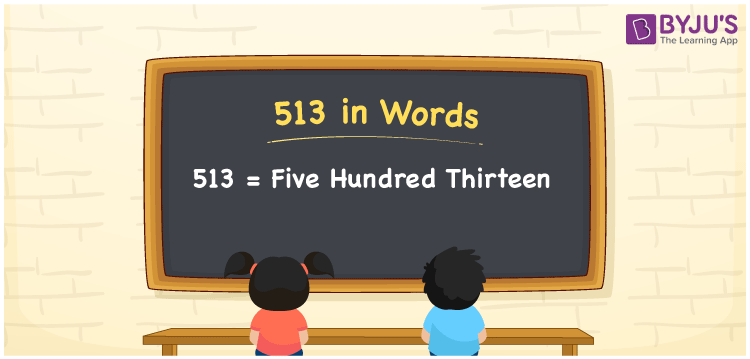# 513 in Words

The number name of 513 in English is “five hundred thirteen”. The place values, such as 3 in one’s place, 1 in ten’s place, and 5 in hundred’s place helps to write the number 513 in words. Also, the number 513 exists between the natural numbers 512 and 514. Also, 513 is a cardinal number

 513 in Words: Five Hundred Thirteen. Five Hundred Thirteen in Numerical Form: 513.

## 513 in English Words## How to Write 513 in Words?

Go through the place value table for the number 513, which is presented below.

 Hundreds Tens Ones 5 1 3

The expanded form of 513 is as follows:

= 5 × Hundred + 1 × Ten + 3 × One

= 5 × 100 + 1 × 10 + 3 × 1

= 500 + 10 + 3

= 513

= Five hundred thirteen

Hence, 513 in words is five hundred thirteen.

513 in words – Five hundred thirteen

Is 513 an odd number? – Yes

Is 513 an even number? – No

Is 513 a perfect square number? – No

Is 513 a perfect cube number? – No

Is 513 a prime number? – No

Is 513 a composite number? – Yes

## Frequently Asked Questions on 513 in Words

Q1

### How to spell 513 in English words?

513 in words is five hundred thirteen.

Q2

### Simplify 500 + 13, and express it in words.

Simplifying 500 + 13, we get 513. Hence, 513 in words is five hundred thirteen.

Q3

### Is 513 a prime number?

No, 513 is not a prime number.